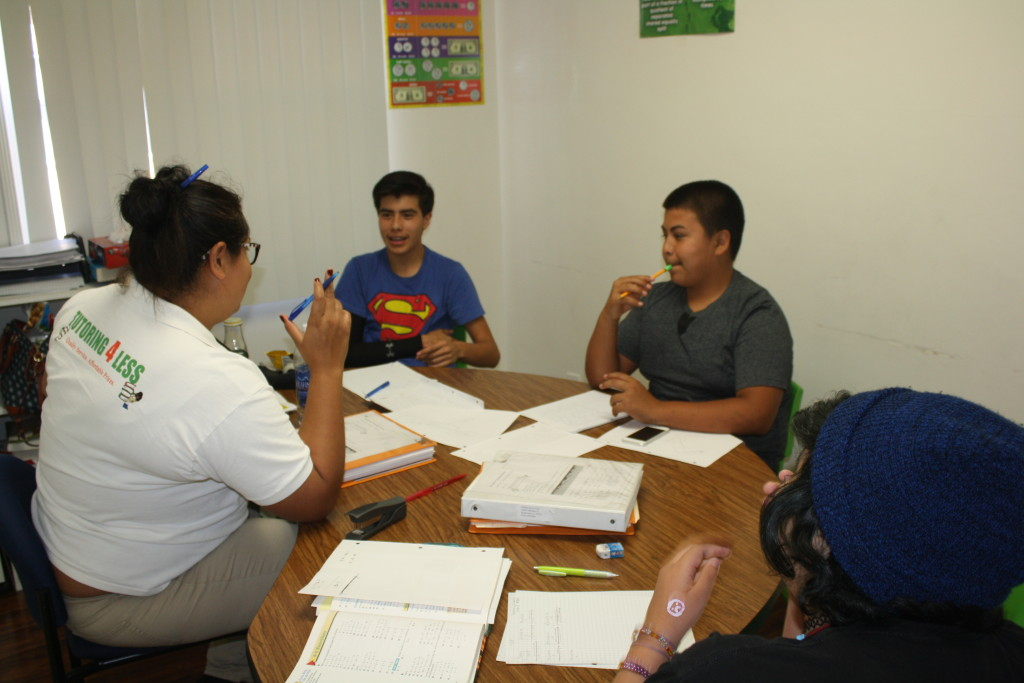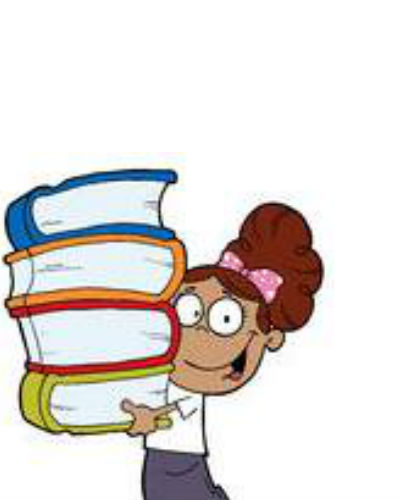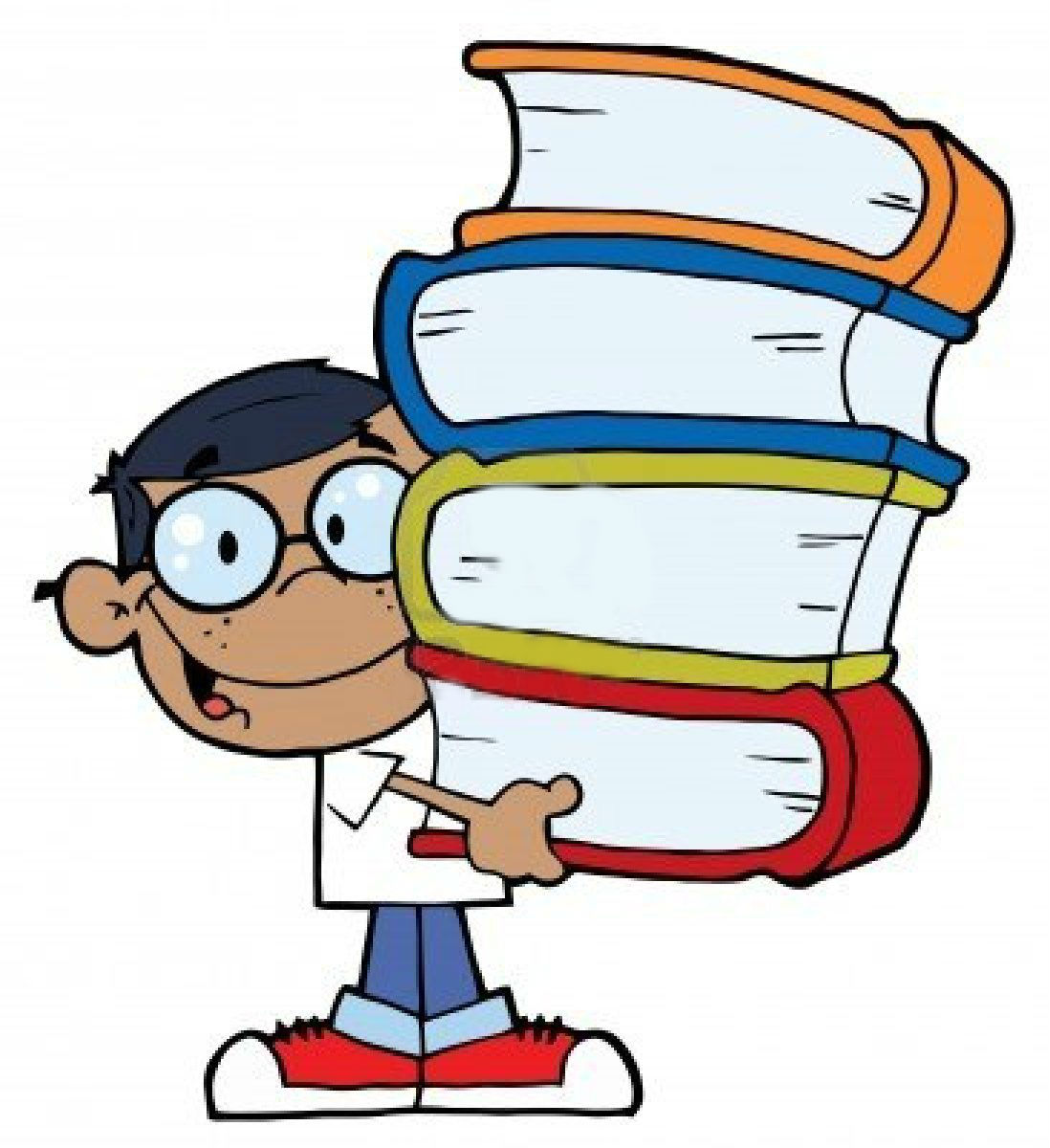#Tutoring 4 Less is the place to go for pre-algebra tutoring for middle school students. We excel at introducing and reinforcing the concepts needed for higher level mathematics. At Tutoring 4 Less we strive to prepare each student with the skills that are crucial to becoming independent learners. By the end of our program students are able to demonstrate a mastery of skills and knowledge in Pre-Algebra.  We want all of our tutored students to recognize the importance of Pre-Algebra in the real world, and to be able to demonstrate confidence and enthusiasm in the classroom.

## Private Pre-Algebra Tutoring for Improved Grades

Our goal is to provide a successful transition from the basic skills learned in elementary mathematics to the advanced mathematical skills needed to succeed in middle school, high school, and beyond. Most students begin their first transitional year of middle school reviewing and relearning a variety of mathematical concepts to reinforce their understanding in the subject matter. Mastery of the fundamental skills at the beginning of their middle school years is essential in preparing students for the subsequent math courses. Pre-Algebra, Algebra, Statistics, and Geometry, are learned as a part of an integrated sequence of courses, with each different subject area receiving emphasis each year. As students progress, they will become ready for high school math classes, and the challenges that they entail.## Online Tutoring For Pre-Algebra

Why choose online tutoring for pre-algebra? In 2020, our expectation of online learning has dramatically changed, and Tutoring 4 Less has adapted its proven programs to an effective online format.

As a parent of a middle schooler, you may have concerns about the effectiveness of pre-algebra tutoring online. The average math class is not an optimal learning environment for many. Not all learning styles mesh well with a noisy, crowded classroom, especially when the subject matter includes algebra and other abstract mathematical principles. Luckily, the math tutors at Tutoring 4 Less are highly experienced working with students that have a variety of educational needs, and can adapt their teaching styles to best fit their needs. We offer online tutoring sessions as an alternative to traditional in-person private tutoring experiences.

## Is Pre-Algebra Hard?

It can be, but not if it is taught and studied sequentially, new skills and concepts building on each other, with respect for the student’s learning style. You don’t build a pyramid from the top, and nor do you teach a pre-teen algebra by throwing advanced concepts at them before they are prepared. By the end of their middle school years, students are expected to be advanced in algebraic notation and reasoning. They should understand how to integrate algebraic concepts and skills with other advanced branches of mathematics. At Tutoring 4 Less we organize these learning goals across five learning branches with clearly defined academic goals.

• Numbers and Operations: Our primary focus is to develop computational fluency with fractions, decimals, and integers. Students are well versed in using the order of operations and how to apply it to problem solving. By the end of 8th grade, students should have a well-developed understanding of algebraic ideas and concepts.
• Problem Solving: Students will be able to make sense of problems and be proficient in explaining and analyzing their meaning. They should be comfortable enough to make conjectures about the form and meaning of the problems and plan a solution pathway rather than just memorize steps and jump into guessing a solution. In most cases, they use their knowledge of algebraic expressions and use linear equations to solve the problems. The ultimate goal of this branch of middle school mathematics is to get students to explain the correlation between equations, verbal descriptions, tables, and graphs.
• Geometry and Measurement: This area is focused on heavily during middle school because it is vitally important.  This branch in the curriculum is directly linked to the ideas learned in all other areas of middle school mathematics, especially algebra and statistics. Students learn about conversions, units, and different objects; both planar and three-dimensional. Students expand on their ability to calculate area, volumes, and perimeters. Students in grade 6 build on their knowledge of area by reasoning about relationships among shapes to determine area, surface area, and volume. They find areas of right triangles, other triangles, and special quadrilaterals by decomposing these shapes, rearranging or removing pieces, and relating the shapes to rectangles. Using these methods, students discuss, develop, and justify formulas for areas of triangles and parallelograms. Students find areas of polygons, and surface areas of prisms and pyramids by deconstructing them into pieces with areas they can determine. They prepare for work on scale drawings and constructions in grade 7 by drawing polygons in the coordinate plane.
• Quantities and Relationships: Students in middle school are introduced to rates and ratios. They are expected to not only solve rate and ratio problems graphically and algebraically, but are encouraged to use the learned concepts and terminology to describe the relationships between the compared quantities. Students in middle school are also introduced to negative integers for the first time. They are expected to understand that positive and negative numbers are used together to describe quantities having opposite directions or values (e.g., temperature above/below zero, elevation above/below sea level, credits/debits, positive/negative electric charge). Students use positive and negative numbers to represent quantities in real-world contexts, explaining the meaning of 0 in each situation.
• Algebra: Algebra is the branch of mathematics that focuses on representing numbers and quantities through variables (letters). Students learn to manipulate symbols and equations to build tables, models, and graphs to outline real world applications. They understand numbers, ways of representing numbers, relationships amongst numbers, and number systems. Algebra reinforces the ability to reason and represent ideas in various forms. Students are encouraged to look for patterns to solve a range of problems by gathering and organizing data to make generalizations about mathematical concepts. They are also taught to analyze mathematical situations and structures using algebraic symbols and functions.